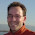## Monday, November 29, 2010

### Multicore Application Programming: Source code

I've just uploaded all the source code to the examples in Multicore Application Programming. About 160 files.

1.Hi, going through your book right now. I was wondering on listing 6.15 why you don't include the counter < 100 check in the critical section. If I change 100 to 10, and run it a bunch of times, I get (for counter < 10):

ThreadID 3580; value = 0, is prime = 1
ThreadID 3580; value = 1, is prime = 1 // 1 is not a prime number ;-)
ThreadID 3580; value = 2, is prime = 1
ThreadID 3580; value = 3, is prime = 1
ThreadID 3580; value = 4, is prime = 0
ThreadID 4112; value = 5, is prime = 1
ThreadID 3580; value = 6, is prime = 0
ThreadID 4112; value = 7, is prime = 1
ThreadID 3580; value = 8, is prime = 0
ThreadID 4112; value = 9, is prime = 0
ThreadID 3580; value = 10, is prime = 0 // whoops :-)

2.Steven,

Thanks for pointing that out. You're quite correct there is a race between the thread checking whether it should exit and then getting the value for the next element.

In writing the code I wasn't worried about the boundary conditions. The most important thing, for me, was avoiding duplicate calculations.

The code as written could over count by the number of threads minus one.

To handle this in a more robust way I'd probably make a local copy of the counter variable, update that using the critical section, and then check for the exit condition before doing the calculation if needed. But there are plenty of other options.

Regards,

Darryl.